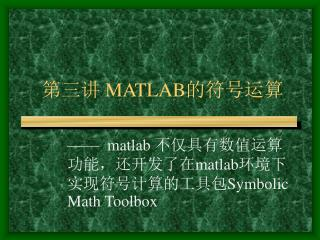DownloadDownload Presentation# 第三讲 MATLAB 的符号运算

Download Presentation## 第三讲 MATLAB 的符号运算

- - - - - - - - - - - - - - - - - - - - - - - - - - - E N D - - - - - - - - - - - - - - - - - - - - - - - - - - -
##### Presentation Transcript

1. 第三讲 MATLAB的符号运算 —— matlab 不仅具有数值运算功能，还开发了在matlab环境下实现符号计算的工具包Symbolic Math Toolbox

2. 符号运算的功能 • 符号表达式、符号矩阵的创建 • 符号线性代数 • 因式分解、展开和简化 • 符号代数方程求解 • 符号微积分 • 符号微分方程

3. 一、符号运算的基本操作 • 什么是符号运算 • 与数值运算的区别 ※数值运算中必须先对变量赋值，然后才能参与运算。 ※符号运算无须事先对独立变量赋值，运算结果以标准的符号形式表达。

4. 特点：  运算对象可以是没赋值的符号变量  可以获得任意精度的解 • Symbolic Math Toolbox——符号运算工具包通过调用Maple软件实现符号计算的。 • maple软件——主要功能是符号运算， 它占据符号软件的主导地位。

5. 2. 符号变量与符号表达式 f = 'sin(x)+5x' f —— 符号变量名 sin(x)+5x—— 符号表达式 ' '—— 符号标识 • 符号表达式一定要用' ' 单引 号括起来matlab才能识别。

6. ' ' 的内容可以是符号表达式，也可以是符号方程。 例： f1='ax^2+bx+c' —— 二次三项式 f2= 'ax^2+bx+c=0' —— 方程 f3='Dy+y^2=1' ——微分方程 ※符号表达式或符号方程可以赋给符号变量，以后调用方便；也可以不赋给符号变量直接参与运算

7. 3.符号矩阵的创建 数值矩阵A=[1,2;3,4] A=[a,b;c,d] —— 不识别 用matlab函数sym创建矩阵（symbolic 的缩写) 命令格式：A=sym('[ ]') ※ 符号矩阵内容同数值矩阵 ※ 需用sym指令定义 ※ 需用' '标识

8. 例如：A = sym('[a , 2*b ; 3*a , 0]') A = [ a, 2*b] [3*a, 0] 这就完成了一个符号矩阵的创建。 注意：符号矩阵的每一行的两端都有方 括号，这是与 matlab数值矩阵的 一个重要区别。

9. 用字符串直接创建矩阵 • 模仿matlab数值矩阵的创建方法 • 需保证同一列中各元素字符串有相 同的长度。 例：A =['[ a,2*b]'; '[3*a, 0]'] A = [ a, 2*b] [3*a, 0]

10.  符号矩阵的修改 a.直接修改 可用、 键找到所要修改的矩阵，直接修改 b.指令修改 • 用A1=sym(A,,,'new') 来修改。 • 用A1=subs(A, 'new', 'old')来修改 • A1=subs(S, 'old' ,'new')

11. 例如：A =[ a, 2*b] [3*a, 0] A1=sym(A,2,2, '4*b') A1 =[ a, 2*b] [3*a, 4*b] A(2,2)='4*b' A3 = [ a, 2*b] [3*a, 4*b] A2=subs(A1, 'c', 'b') A2 =[ a, 2*c] [3*a, 4*c]

12.  符号矩阵与数值矩阵的转换 • 将数值矩阵转化为符号矩阵 函数调用格式：sym(A) A=[1/3,2.5;1/0.7,2/5] A = 0.3333 2.5000 1.4286 0.4000 sym(A) ans = [ 1/3, 5/2] [10/7, 2/5]

13. 将符号矩阵转化为数值矩阵 函数调用格式： numeric(A) A = [ 1/3, 5/2] [10/7, 2/5] numeric(A) ans = 0.3333 2.5000 1.4286 0.4000

14. 二、符号运算 • 符号矩阵运算 数值运算中，所有矩阵运算操作指 令都比较直观、简单。例如：a=b+c; a=a*b ；A=2*a^2+3*a-5等。 而符号运算就不同了，所有涉及符 号运算的操作都有专用函数来进行

15. 符号矩阵运算的函数： symadd(a,d) —— 符号矩阵的加 symsub(a,b) —— 符号矩阵的减 symmul(a,b) —— 符号矩阵的乘 symdiv(a,b) —— 符号矩阵的除 sympow(a,b) —— 符号矩阵的幂运算 symop(a,b) —— 符号矩阵的综合运算

16. 例1：f= '2*x^2+3*x-5'; g= 'x^2+x-7'; h= symadd(f,g) h= 3*x^2+4*x-12 例2：f='cos(x)';g= ' sin(2*x)'; symop(f,'/',g,'+',f,'*',g) ans = cos(x)/sin(2*x)+cos(x)*sin(2*x)

17. 例1：f= 2*x^2+3*x-5; g= x^2+x-7; >> syms x >> f=2*x^2+3*x-5; g= x^2+x-7; >> h=f+g h = 3*x^2+4*x-12 例2：f=cos(x);g= sin(2*x); >> syms x >> f=cos(x);g=sin(2*x); >> f/g+f*g ans = cos(x)/sin(x)+cos(x)*sin(x)

18. 符号运算函数： symsize —— 求符号矩阵维数 charploy —— 特征多项式 determ —— 符号矩阵行列式的值 eigensys —— 特征值和特征向量 inverse —— 逆矩阵 transpose —— 矩阵的转置 jordan ——约当标准型 simple —— 符号矩阵简化

19. 2. 任意精度的数学运算 在symbolic中有三种不同的算术运算： • 数值类型 matlab的浮点算术运算 • 有理数类型 maple的精确符号运算 • vpa类型 maple的任意精度算术 运算

20. 浮点算术运算 1/2+1/3 －－(定义输出格式format long) ans = 0.83333333333333 • 符号运算 sym(1/2)+(1/3) ans = 5/6 －－精确解

21. 任意精度算术运算 digits(n) —— 设置可变精度，缺省16位 vpa(x,n) —— 显示可变精度计算 digits(25) vpa(1/2+1/3) ans = .8333333333333333333333333

22. vpa(5/6,40) ans = .8333333333333333333333333333333333333333 a=sym('[1/4,exp(1);log(3),3/7]') a = [ 1/4,exp(1)] [log(3), 3/7] vpa(a,10) ans = [.2500000000, 2.718281828] [1.098612289, .4285714286]

23. 3. 符号微积分与积分变换 • diff(f) — 对缺省变量求微分 • diff(f,v) — 对指定变量v求微分 • diff(f,v,n) —对指定变量v求n阶微分 • int(f) — 对f表达式的缺省变量求积分 • int(f,v) — 对f表达式的v变量求积分 • int(f,v,a,b) — 对f表达式的v变量在（a,b) 区间求定积分

24. int（'被积表达式'，'积分变量'，'积分上限'，'积分下限'）—— 定积分 ——缺省时为不定积分 mtaylor(f,n) —— 泰勒级数展开 ztrans(f) —— Z变换 Invztrans(f) —— 反Z变换 Laplace(f) —— 拉氏变换 Invlaplace(f) —— 反拉氏变换 fourier(f) —— 付氏变换 Invfourier(f) —— 反付氏变换

25. 例1.计算二重不定积分 F=int(int('x*exp(-x*y)','x'),'y') F= 1/y*exp(-x*y) 例2.计算 f='x*exp(-x*10)'的Z变换 F=ztrans(f) F= z*exp(-10)/(z-exp(-10))^2

26. >> syms x y >> F=int(int(x*exp(-x*y),x),y) F = 1/y*exp(-x*y) >> syms x >> f=x*exp(-x*10); >> F=ztrans(f) >> F=ztrans(x*exp(-x*10); F = z*exp(-10)/(z-exp(-10))^2

27. eAt = 例3. 计算指数函数eAt。 用拉氏反变换法计算eAt的公式为： eAt = L-1[(SI-A)-1] 系统矩阵A= 结果：

28. >> a=[0 1;-2 -3]; >> syms s >> b=(s*eye(2)-a) b = [ s, -1] [ 2, s+3] >> B=inv(b) [ (s+3)/(s^2+3*s+2), 1/(s^2+3*s+2)] [ -2/(s^2+3*s+2), s/(s^2+3*s+2)]

29. >>b11=ilaplace(sym(b,1,1));b(1,1)=b11; >> b12=ilaplace(sym(b,1,2));b(1,2)=b12; >> b21=ilaplace(sym(b,2,1));b(2,1)=b21; >> b22=ilaplace(sym(b,2,2));b(2,2)=b22; >> b b = [ -exp(-2*t)+2*exp(-t), exp(-t)-exp(-2*t)] [ -2*exp(-t)+2*exp(-2*t), 2*exp(-2*t)-exp(-t)]

30. matlab符号运算能够解一般的线性方程、非线性方程及一般的代数方程、代数方程组。当方程组不存在符号解时，又无其他自由参数，则给出数值解。 命令格式： solve(f) —— 求一个方程的解 Solve(f1,f2, …fn) —— 求n个方程的解 4.符号代数方程求解

31. 例1. f = ax2+bx+c 求解 f='a*x^2+b*x+c'; • solve(f) —— 对缺省变量x求解 ans = [1/2/a*(-b+(b^2-4*a*c)^(1/2))] [1/2/a*(-b-(b^2-4*a*c)^(1/2))] 计算机 格式 一般格式

32. solve(f , 'b' ) —— 对指定变量b求解 • ans = • -(a*x^2+c)/x 例2. 符号方程cos(x)=sin(x) tan(2*x)=sin(x)求解 f1=solve('cos(x)=sin(x)'), f1 = 1/4*pi

33. f2=solve('tan(2*x)=sin(x)') f2 = matlab4.2的解 [ 0] [acos(1/2+1/2*3^(1/2))] [acos(1/2 -1/2*3^(1/2))] f3= matlab4.2的解 [ 0] [ pi] [ atan(1/2*(-2*3^(1/2))^(1/2),1/2+1/2*3^(1/2))] [ atan(-1/2*(-2*3^(1/2))^(1/2),1/2+1/2*3^(1/2))] [ atan(1/2*2^(1/2)*3^(1/4)/(1/2-1/2*3^(1/2)))+pi] [ -atan(1/2*2^(1/2)*3^(1/4)/(1/2-1/2*3^(1/2)))-pi]

34. numeric(f2) ans = 0 0 + 0.8314i 1.9455 matlab4.2与6.1的对比 numeric(f3) ans = 0 3.1416 0 + 0.8314i 0 - 0.8314i 1.9455 -1.9455

35. 例3. 解方程组 x+y+z=1 x-y+z=2 2x-y-z=1 g1='x+y+z=1',g2='x-y+z=2',g3='2*x-y-z=1' f=solve(g1,g2,g3) f=solve('x+y+z=1','x-y+z=2','2*x-y-z=1') f = z = 5/6, y = -1/2, x = 2/3

36. f=solve('x+y+z=1','x-y+z=2','2*x-y-z=1') f = x: [1x1 sym] f.x ans =2/3 y: [1x1 sym] f.y ans =-1/2 z: [1x1 sym] f.z ans =5/6 [x,y,z]=solve('x+y+z=1','x-y+z=2','2*x-y-z=1') x = 2/3 y =-1/2 z =5/6

37. 5. 符号微分方程求解 —— 用一个函数可以方便地得到微 分方程的符号解 符号微分方程求解指令：dsolve 命令格式：dsolve(f,g) • f —— 微分方程，可多至12个微分方程的求 解；g为初始条件 • 默认自变量为 'x',可任意指定自变量't', 'u'等 • 微分方程的各阶导数项以大写字母D表示

38. y的一阶导数—— Dy 或 y的二阶导数—— D2y 或 y的 n 阶导数—— Dny [y1,y2…]=dsolve(x1,x2,…xn) —— 返回 微分方程的解

39. 一阶微分方程 dsolve('Dx=y','Dy=x','x(0)=0','y(0)=1') ans = x(t) = sin(t), y(t) = cos(t) 二阶微分方程 dsolve('D2y=-a^2*y','y(0)=1','Dy(pi/a)=0') ans = cos(a*x)

40. 例3. y=dsolve('D2y+2*Dy+2*y=0','y(0)=1','Dy(0)=0') ans = exp(-x)*cos(x)+exp(-x)*sin(x) ezplot(y) —— 方程解y(t)的时间曲线图 求该方程的解

41. 三、maple函数——符号运算的扩展 maple——是专门进行数学运算的软件工具， 具有超强的符号运算能力，提供了 几乎包括所有数学领域的专用函数 matlab——依赖于maple的内核与函数库，扩 展了自己的符号运算功能。 matlab还设计了对maple库函数的调用功能 使得已有的maple数学功能，可以扩充matlab 中,作为自身符号运算能力的扩展。

42. 1. maple内核访问函数 可以访问maple内核的matlab函数: maple ——— 访问maple内核函数 mapleinit —— maple函数初始化 mpa ———— maple函数定义 mhelp ——— maple函数帮助命令 procread —— maple函数程序安装

43. . maple 的调用格式 maple('表达式'） —— 将表达式送至maple内核， 返回符号表达式结果。 maple （'函数'，变量1，变量2） ——调用maple函数，传递给定 变量。

44. 例1. 展开5阶 bernoulli 多项式，计算 x=3 时bernoulli 数。 a=maple('bernoulli(5,x)') a = -1/6*x+5/3*x^3+x^5-5/2*x^4 a=maple('bernoulli(5,3)') a = 85

45. 例2. 化简三角函数式sin2x+cos2x a=maple('simplify(sin(x)^2+cos(x)^2);') a = 1 例4. 求f(t)=e-3tsint的拉式变换 f=maple('laplace(exp(-3*t)*sin(t),t,s);') f = 1/((s+3)^2+1)

46. 例4. 寻找二次多项式的完全平方 f (x) = x2+2x+2 a=maple('completesquare(x^2+2*x+2)') a = completesquare(x^2+2*x+2) 将工具包装入内存 maple('with(student);') a=maple('completesquare(x^2+2*x+2)') a = (x+1)^2+1